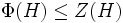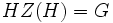# Extraspecial and critical implies whole group

## Statement

Suppose$G$ is a Group of prime power order (?), and$H$ is a Critical subgroup (?) of$G$ that is also an Extraspecial group (?). Then,$H = G$.

## Definitions used

### Critical subgroup

Further information: critical subgroup

A characteristic subgroup$H$ of a finite$p$-group$G$ is termed critical if it satisfies the following conditions:

1.$\Phi(H) \le Z(H)$, viz the Frattini subgroup is contained inside the center (i.e.,$H$ is a Frattini-in-center group).
2.$[G,H] \le Z(H)$ (i.e.,$H$ is a commutator-in-center subgroup of$G$).
3.$C_G(H)= Z(H)$ (i.e.,$H$ is a self-centralizing subgroup of$G$).

## Facts used

1. Extraspecial commutator-in-center subgroup is central factor

## Proof

Given: A finite$p$-group$G$, a critical subgroup$H$ that is also extraspecial.

To prove:$H = G$.

Proof: By point (2) of the definition of critical subgroup,$H$ is a commutator-in-center subgroup of$G$. Combining this with fact (1) yields that$H$ is a central factor of$G$. Thus,$HC_G(H) = G$.

Point (3) of the definition of critical subgroup says that$C_G(H) = Z(H)$, so$HZ(H) = G$, so$H = G$, completing the proof.